# How to Translate Word Problems Into EquationsMath is complicated enough when trying to make sense of numbers alone, and even more so when words are thrown into the mix. In this useful Math with Margo tutorial, she’ll show students how to convert word problems into algebra terms and then find an easy solution.

Follow along step-by-step, and your student will understand algebra terms and how they fit into complex math problems.

## Key Vocabulary

Before we begin, let’s touch on some important vocabulary. What is a variable? An expression? Here’s a quick refresher before we dive into today’s problem.

Variable: In algebra, a variable like x or y symbolizes an unknown value.

Expression: A mathematical expression is a group of numbers, operators, and symbols. Expressions can include addition, multiplication, subtraction, and division.

Terms: A term is a single mathematical expression: one number, operator, or symbol.

## How to Translate Word Problems Into Equations

Let's go through an example problem to get some practice translating word problems into equations.

Problem: A person exchanges 390 pennies for quarters, dimes, and nickels. The number of dimes in the exchange was twice the number of quarters and the number of nickels was twice the number of dimes. How many quarters were in the exchange?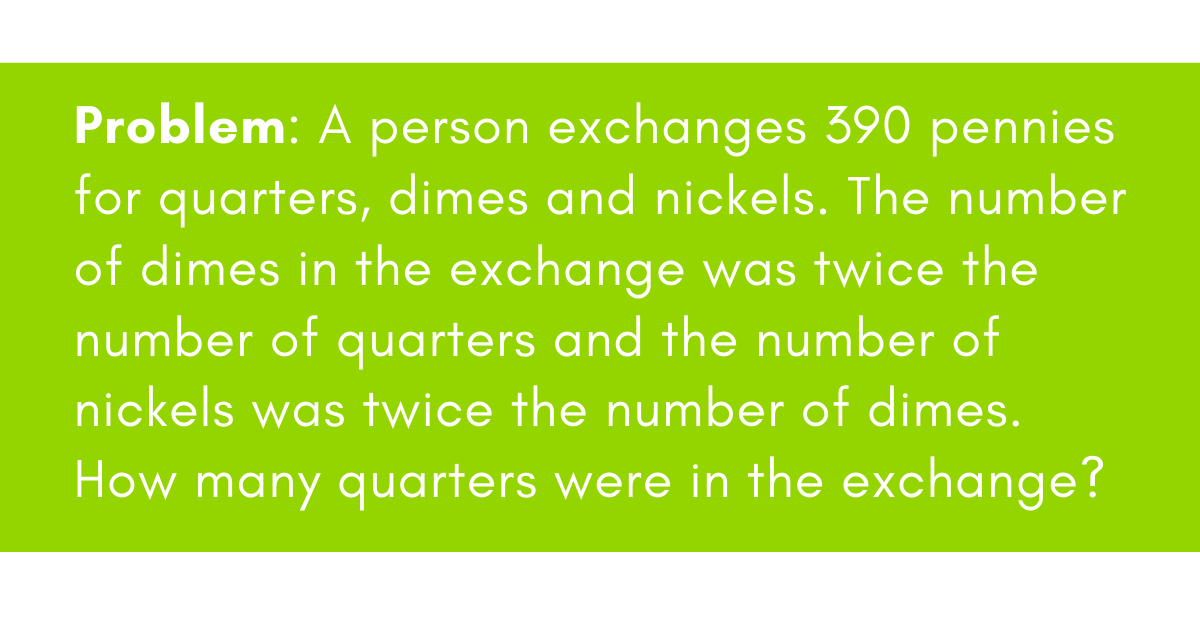Confused? Never fear! Word problems like this seem complicated at first, but with Margo’s method, soon you’ll understand the key parts.

### Step One: Highlight Important Parts of the Text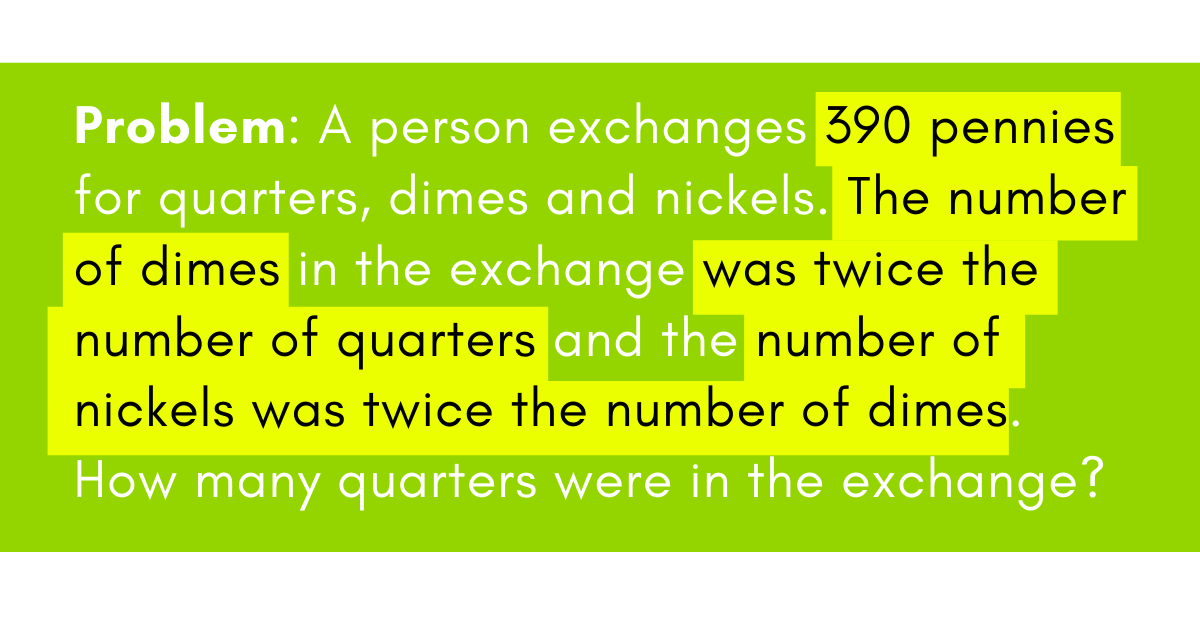### Step Two: Establish your variables

• q represents the # of quarters
• d represents the # of dimes
• n represents the # of nickels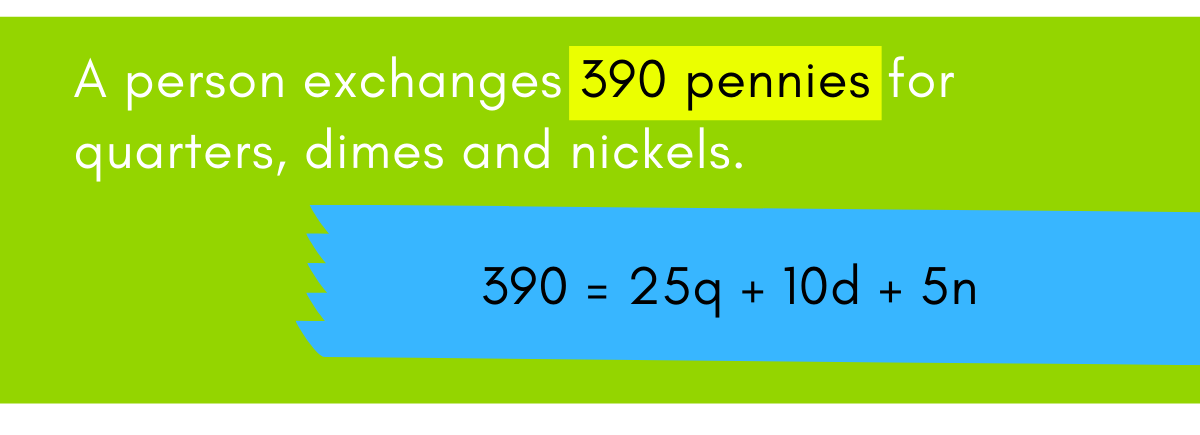### Step Three: Rewrite the Problem as an Equation

Since we have three equations and three variables, we can use substitution to solve for a variable. Which variable is in each equation?

That’s right, d is.

d = 2q needs to be written as “q =” so we can replace the q in the first equation with something in terms of d. If we divide both sides by 2, we are left with d/2 = q.

n = 2d is already in the form we want. We can substitute n in the first equation with 2d, since they are equal in value.

390 = 25q + 10d + 5n
d = 2q   ⇒ d/2 = q
n = 2d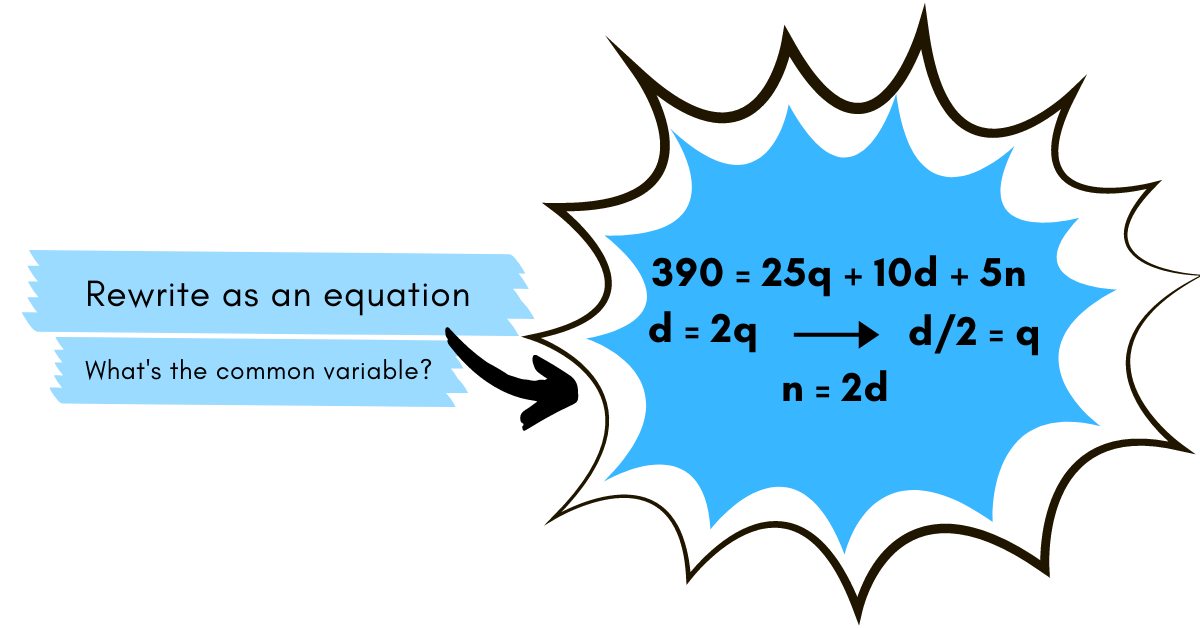### Step Four: Substitute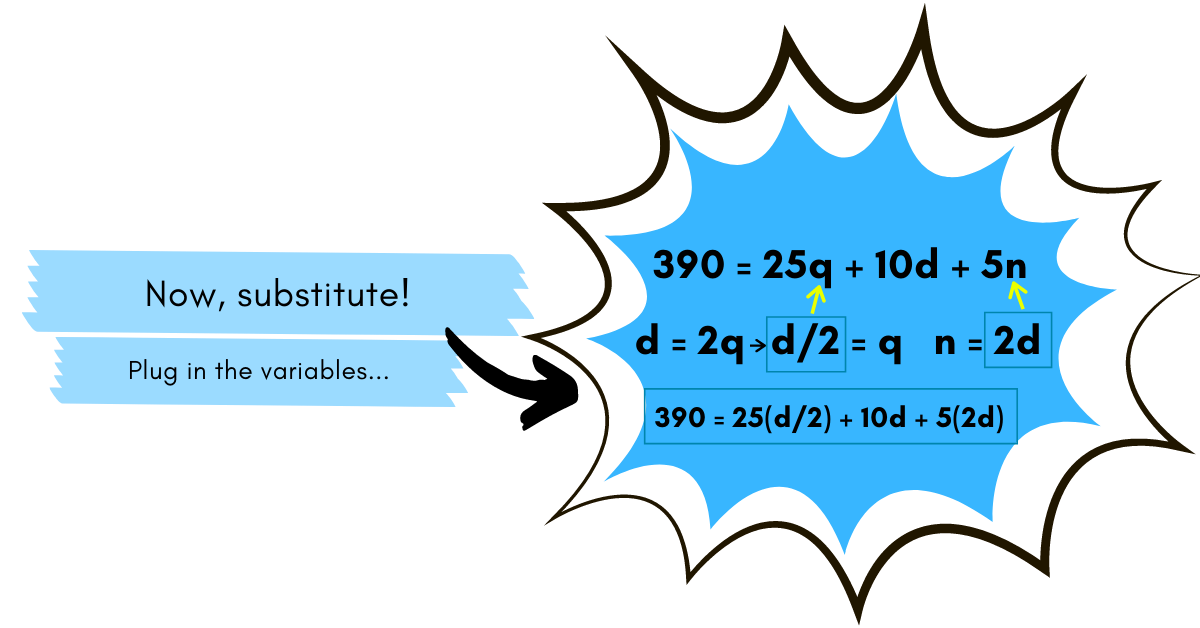Now we have one equation with one variable, and we can simplify it to solve out for d.

### Step Five: Simplify and Combine Terms

When a number is pushed up against a variable it implies multiplication, so to get the d variable by itself. We need to do the opposite operation, so divide both sides by 32.5.

Remember, "d" represents the number of dimes, so there were 12 dimes in the exchange.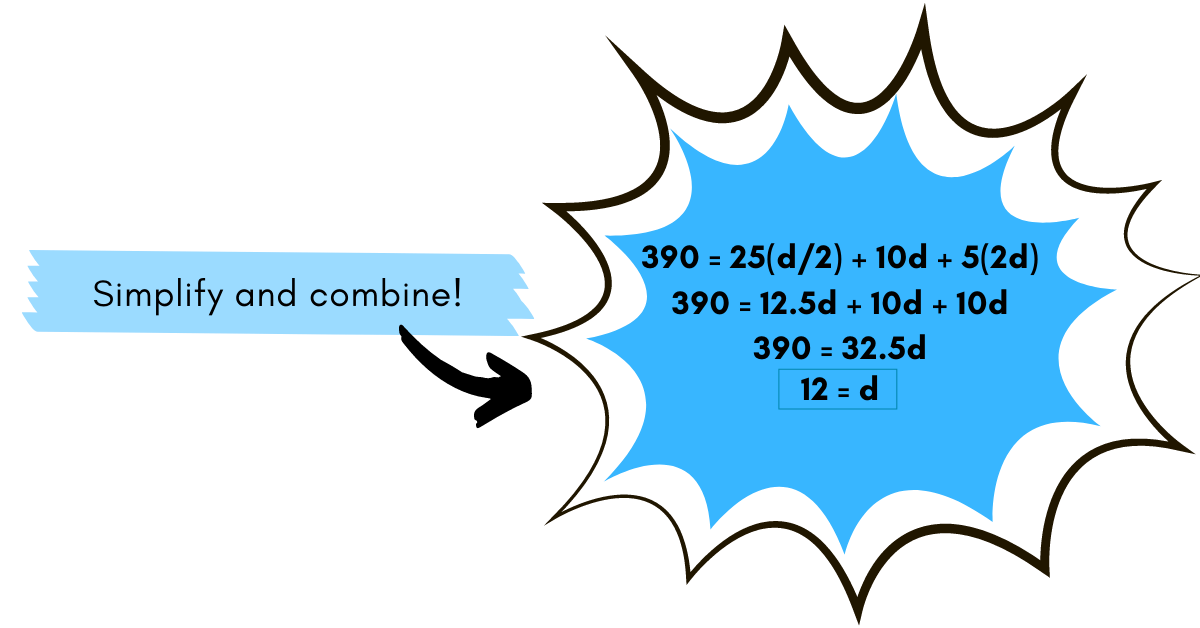### Step Six: Divide Each Side of the Equation

Since we are ultimately trying to find out how many quarters were in the exchange, we can use the original equation we set up that has a relationship between the number of dimes and the number of quarters.

We know that d=12, so we can substitute d for 12 and then solve for q.

Divide both sides of the equation by 2 to get q by itself.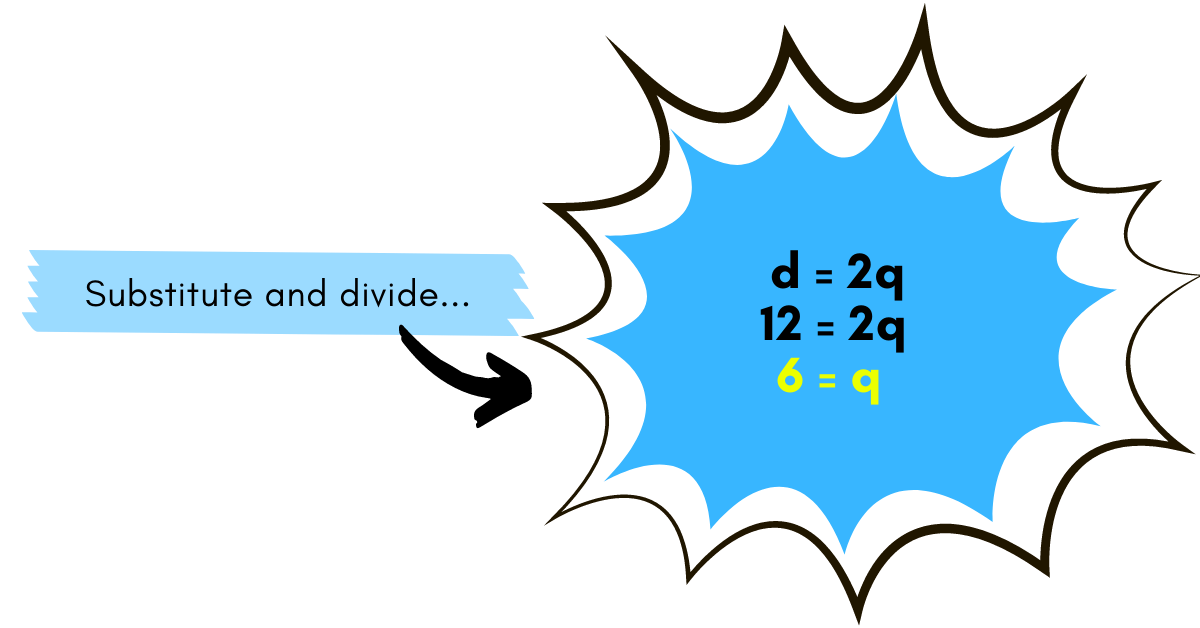### Step Seven: Solve

Therefore, our final answer is that there were 6 quarters in the exchange!

As the above hopefully shows, math doesn’t need to be a headache; in fact, there are many ways to make math fun! If your child has an aptitude for math, but could use a challenge, a math competition or game could be just the motivator they need.

If math class isn't not your student’s cup of tea, there are excellent resources to support their learning out there, like this recent post about fascinating math facts and apps sure to pique the interest of reluctant mathematicians.

Plus, Margo’s math expertise spans other tutorials in solving for x, converting decimals into fractions, and some helpful advice for studying for that next math test or quiz. Our math tips & resources page is a great place to bring the "M" in STEM front and center.

For more personalized support, iD Tech’s top-notch instructors offer one-on-one online math tutoring in subjects ranging from geometry to pre-algebra and statistics to help kids achieve their goals in math.Virginia started with iD Tech at the University of Denver in 2015 and has loved every minute since then! A former teacher by trade, she has a master's in education and loves working to embolden the next generation through STEM. Outside the office, you can usually find her reading a good book, struggling on a yoga mat, or exploring the Rocky Mountains.

Featured Posts

Categories

Authors